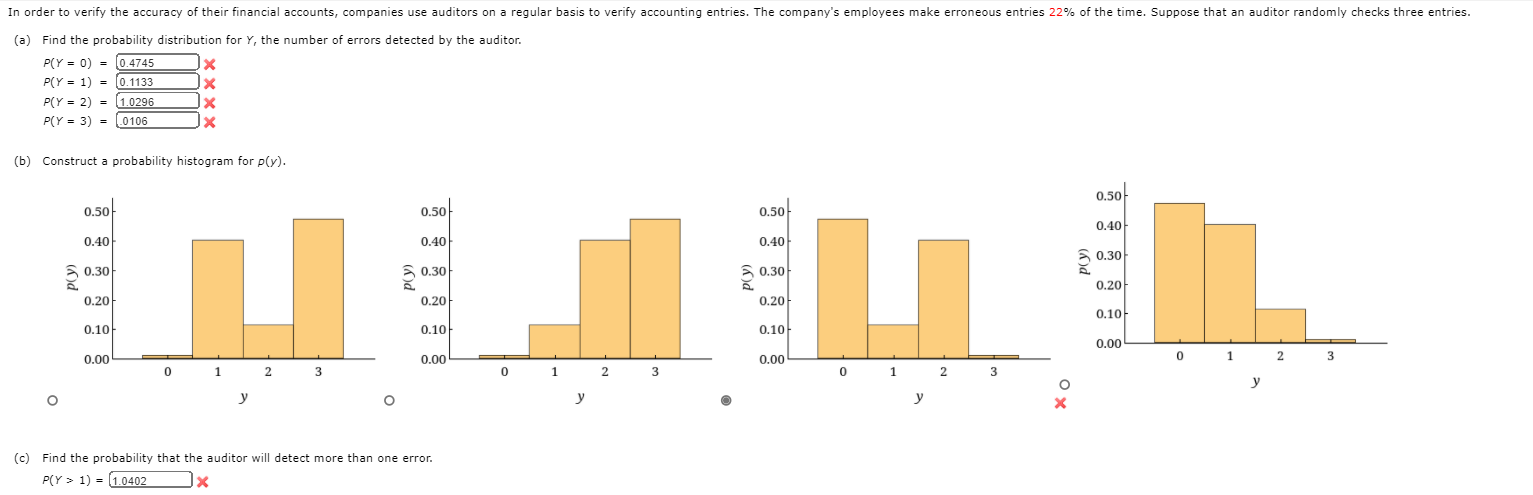# Question Solved1 AnswerIn order to verify the accuracy of their financial accounts, companies use auditors on a regular basis to verify accounting entries. The company's employees make erroneous entries 22% of the time. Suppose that an auditor randomly checks three entries. (a) Find the probability distribution for Y, the number of errors detected by the auditor. P(Y=0)= P(Y=1)= P(Y=2)= P(Y=3)= (b) Construct a probability histogram for p(y). (c) Find the probability that the auditor will detect more than one error. In order to verify the accuracy of their financial accounts, companies use auditors on a regular basis to verify accounting entries. The company's employees make erroneous entries 22% of the time. Suppose that an auditor randomly checks three entries. (a) Find the probability distribution for y, the number of errors detected by the auditor. PCY = 0) = 0.4745 X PCY = 1) = 0.1133 JX PCY = 2) = 1.0296 x PCY = 3) = .0106 x (b) Construct a probability histogram for ply). 0.50 0.50 0.50 0.50 0.40 0.40 0.40 0.40 0.30 0.30 50.30 0.30 ру ру ру. 0.20 0.20 0.20 0.20 0.10: 0.10 0.10 0.10 0.00 0.00 0.00 0.00 0 1 2 3 0 1 2 3 0 1 2 3 0 1 2 3 у у o у o у X (c) Find the probability that the auditor will detect more than one error. P(Y > 1) = 1.0402 xREZLWW The Asker · Probability and Statistics

In order to verify the accuracy of their financial accounts, companies use auditors on a regular basis to verify accounting entries. The company's employees make erroneous entries 22% of the time. Suppose that an auditor randomly checks three entries. (a) Find the probability distribution for Y, the number of errors detected by the auditor.

P(Y=0)=

P(Y=1)=

P(Y=2)=

P(Y=3)=

(b)

Construct a probability histogram for p(y).

(c)

Find the probability that the auditor will detect more than one error.Transcribed Image Text: In order to verify the accuracy of their financial accounts, companies use auditors on a regular basis to verify accounting entries. The company's employees make erroneous entries 22% of the time. Suppose that an auditor randomly checks three entries. (a) Find the probability distribution for y, the number of errors detected by the auditor. PCY = 0) = 0.4745 X PCY = 1) = 0.1133 JX PCY = 2) = 1.0296 x PCY = 3) = .0106 x (b) Construct a probability histogram for ply). 0.50 0.50 0.50 0.50 0.40 0.40 0.40 0.40 0.30 0.30 50.30 0.30 ру ру ру. 0.20 0.20 0.20 0.20 0.10: 0.10 0.10 0.10 0.00 0.00 0.00 0.00 0 1 2 3 0 1 2 3 0 1 2 3 0 1 2 3 у у o у o у X (c) Find the probability that the auditor will detect more than one error. P(Y > 1) = 1.0402 x
More
Transcribed Image Text: In order to verify the accuracy of their financial accounts, companies use auditors on a regular basis to verify accounting entries. The company's employees make erroneous entries 22% of the time. Suppose that an auditor randomly checks three entries. (a) Find the probability distribution for y, the number of errors detected by the auditor. PCY = 0) = 0.4745 X PCY = 1) = 0.1133 JX PCY = 2) = 1.0296 x PCY = 3) = .0106 x (b) Construct a probability histogram for ply). 0.50 0.50 0.50 0.50 0.40 0.40 0.40 0.40 0.30 0.30 50.30 0.30 ру ру ру. 0.20 0.20 0.20 0.20 0.10: 0.10 0.10 0.10 0.00 0.00 0.00 0.00 0 1 2 3 0 1 2 3 0 1 2 3 0 1 2 3 у у o у o у X (c) Find the probability that the auditor will detect more than one error. P(Y > 1) = 1.0402 x